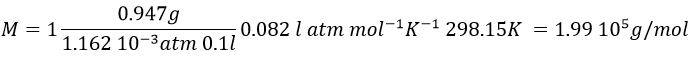# Chapter 4e: Phase diagram

The state of compounds depends on the temperature and on the pressure. We map the states of the matter on the diagram of phases. The pressure is put as ordinate and the temperature as abscise.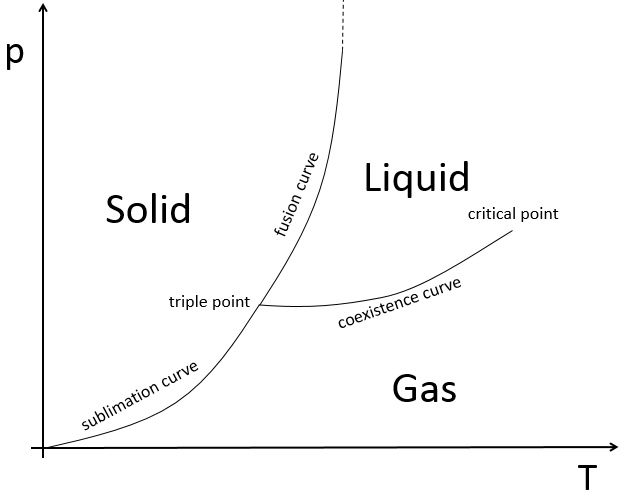The states are separated by full lines, the curves of transition of phase.

The curve between the liquid and the solid is called the curve of fusion.

The curve between the liquid and the gas is called the curve of vaporisation/coexistence.

The curve between the solid and the gas is called the curve of sublimation.

Curve of vaporisation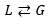In a closed system containing a liquid and a gas, there is an equilibrium between the two phases. The gas becomes liquid at a given speed vGL and the liquid becomes gas at a given speed vLG. The equilibrium is reached when those speeds are equal.

The liquid becomes a gas if the molecules have a kinetic energy large enough. If this energy is not large enough, the molecules remain liquid because the interactions between the molecules make it more favourable. The kinetic energy can be provided by heating or excitation. Typically, we have to heat water to make it boil.

The other reaction, from a gas to a liquid is favourable but is not complete because of the entropy. The entropy is an expression of the disorder in the system. The second law of thermodynamics (the thermodynamics will be explained in its own chapter) imposes that the universe evolves towards a greater disorder. It means that the sum of the variations of entropy inside and outside the system has to be positive.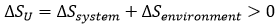There is thus a competition between the increase of entropy and the decrease of the energy in the system.

The free enthalpy of Gibbs represents/reflects this competition: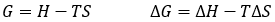The Gibbs free enthalpy is thus a thermodynamic potential taking into account the implications of the system on its environment.

The vapour pressure in the system at the equilibrium (also called the pressure of Clausius-Clapeyron) is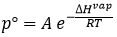The entropic terms are present in the pre-exponential constant and the enthalpy is in the exponential term. As the temperature increases, the vapour pressure increases exponentially. Experimentally, we can find the enthalpy of vaporisation by plotting the curve ln p° vs 1/T.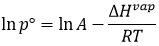The slope of the curve is –ΔH/R. The value of ΔHvap depends on the interactions between the molecules. Strong interactions mean that the molecules are not easily getting away from each other and that the vaporisation requires more energy, i.e. ΔHvap is large and p° is small. It is logical: if the molecules don’t easily vaporise, there are not a lot of molecules in the gas and the pressure of the vapour is thus low.

In the cyclohexane, there are barely no interactions between the molecules and its ΔHvap is small. The cyclohexane is a volatile liquid.

The boiling temperature of a liquid is the temperature at which the vapour pressure of a liquid is equal to the atmospheric pressure. For the water, this temperature is 373K (100°C) if the atmospheric pressure is 1 atm. In altitude, the atmospheric pressure decreases and so does the boiling temperature. For example, the boiling temperature is 363K (90°C) at an altitude of 3000m. In a pressure cooker, the pressure is greater than 1 atm and the water boils at a temperature larger than 100°C.

Curve of sublimation

The sublimation is the transition of phase between a solid and a gas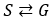We can define a vapour pressure for solids too, but it is much lower than for a liquid because interactions in a solid are stronger than in a liquid. The equation is similar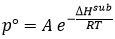But the constant is different and ΔHsub>>ΔHvap, for the exact same reasons.

Curve of fusion

The fusion is the transition of state from a solid to a liquid.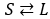We can also define a pressure such as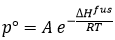The enthalpy of fusion is very small and the curve is almost a right vertical line. In general, the slope is positive, meaning that when a liquid becomes solid (by decreasing its temperature for example), its volume decreases. It is however not always true.

A particular case is the water: the volume of 1kg of water is smaller than the volume of 1kg of ice. It is a tip of the barmen to decrease the volume of drink that they give to their clients but it is also what allow us to ice-skate:

When we skate, all our weight is applied on a very small surface. The ice at this place instantly becomes liquid because of this pressure. If the ice did not change into liquid, the friction between our skates and the ice would be much larger, as it is in general the case between two solids. The small puddle of water that is formed lubricates the ice and allow us to slide with almost no friction. Because the volume of water was smaller than the volume of ice, the water remains in the small hole that our skates dug in the ice. After our passage, the water turns back into ice at the same place where we skated. If the volume was the water was larger than the one of the ice, the puddle would extend near the skates and deform the surface of the ice. If it was the case, in a skating rink, the surface of the ice would not remain flat for a very long time.

The carbon dioxide in an extinguisher is in a liquid state, near the triple point. When we use the extinguisher, the pressure drops suddenly. We should thus expect a gas outside of the tube. However, there is a detente of the gas during the process (moving from a narrow space to a large space) that involves a decrease of the temperature, making the gas become a solid.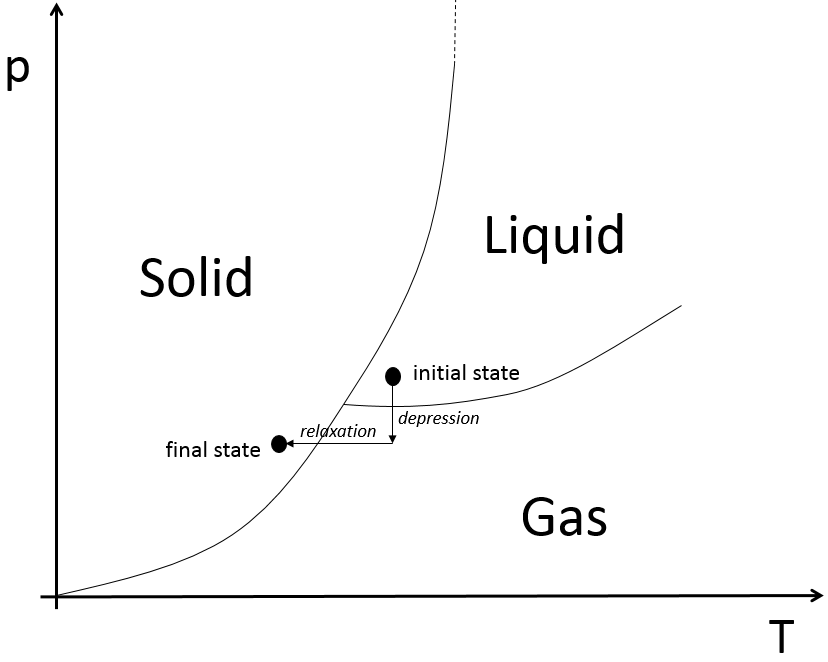Variance

It is the number of degrees of freedom that characterise a state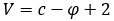Where c is the number of constituents of the matter and φ is the number of phases. Let’s take a look at different points on the phase diagram

• in the middle of a phase: V=1-1+2=2: we can modify the pressure or the temperature without transition of state.
• on a curve: V=1-2+2=1: if we want to modify the pressure or the temperature of the matter without transition of state, the other parameter (T or p) has to be fixed.
• on the triple point: V=1-3+2=0: if we want to keep the matter in this state, we can’t change the conditions of temperature and of pressure.

At the triple point, the enthalpy of sublimation equals the sum of the enthalpies of vaporisation and of fusion. The triple point for the water is at t=273,16K and p=0.006atm.

Another remarkable point is the critical point:

After this point, the liquid and gaseous phases become indistinguishable. In water, the critical point occurs at around 647 K and 218 atm.

In the vicinity of the critical point, the physical properties of the liquid and the vapour change dramatically, with both phases becoming ever more similar. For instance, liquid water under normal conditions is nearly incompressible, has a low thermal expansion coefficient, has a high dielectric constant, and is an excellent solvent for electrolytes. Near the critical point, water becomes compressible, expandable, a poor dielectric, a bad solvent for electrolytes, and prefers to mix with nonpolar gases and organic molecules.

At the critical point, only one phase exists. The heat of vaporization is zero.

Above the critical point (T>Tc and p>pc) there is a state of matter that is continuously connected, without transition of phase, with both the liquid and the gaseous state: supercritical fluid. Such a fluid has usually properties between those of a gas and a liquid.

Case of mixtures

In a solution, the elements of the mixture don’t necessarily have the same boiling point. The element of lower boiling point will evaporate faster than the other components of the mixture. If we consider a binary mixture, we can plot the composition of the melange in function of the temperature on a diagram shown underneath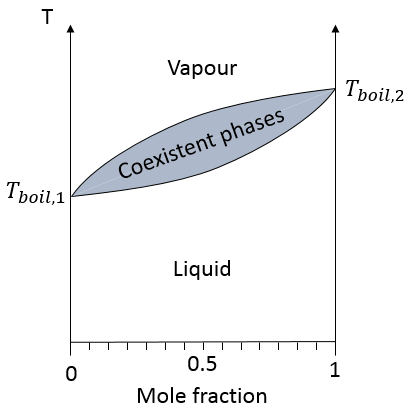On this diagram, the temperature is reported on the ordinate and the mole fraction χ of the second component of the melange is set as abscise. At χ=0, the solution is a pure solution of the solute 1, and the boiling temperature of the solution is Tboil,1. At χ=1, the solution is a pure solution of the solute 2, and the boiling temperature of the solution is Tboil,2.  In between, the solution is a mixture of the two components. In this case, we considered a solution in which the solute 1 has a lower boiling temperature than the solute 2. If we increase the proportion of the solute 2, the boiling temperature of the mixture increases. This increase is not linear and one can see that there is an area in which both vapour and liquid coexist. If you look at a given temperature T’, the composition of the liquid and of the vapour is different: the composition of the vapour is composed by a larger fraction of the most volatile solute than the liquid.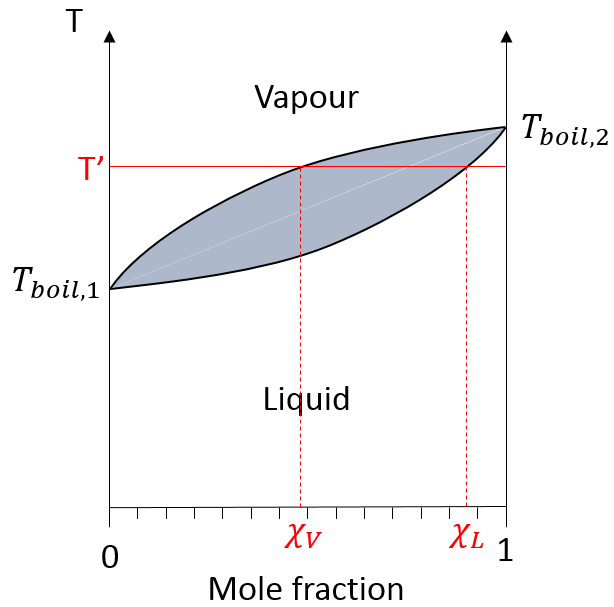The molar fractions of the melange can be determined with the law of Raoul. The total pressure of an ideal mix is the sum of the partial pressures of the gases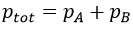The partials pressures are proportional to the mole fraction of the compound in the solution χLA multiplied by the vapour pressure of the pure compound p°A: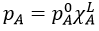The total pressure is thus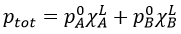Given that the sum of the mole fractions in solution is equal to 1, i.e. χLA+ χLA=1, we find the mole fraction of one species from the pressures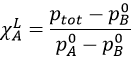Moreover, this allows us to connect the vapour and liquid mole fractions together by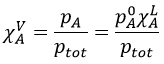Distillation

The distillation is a separation process based on the difference of boiling temperature of different solutes in a solution. Basically, if the initial solution has a mole fraction χL, we can boil it at the temperature T’. The goal is to collect the vapour and to liquefy it. The collected solution has a composition χV. We can keep doing this multiple times to obtain a virtually pure solution of the solute 1.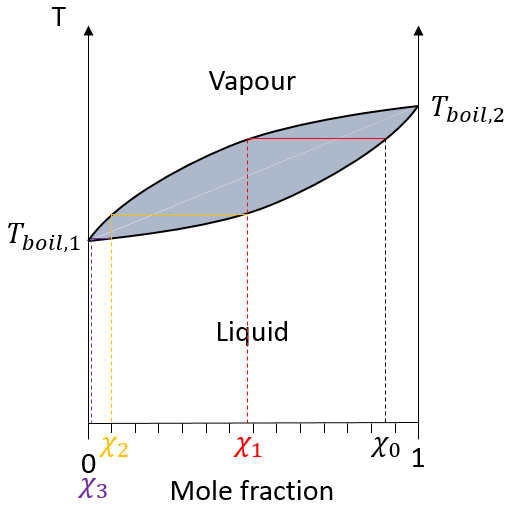In practical, multiple distillations are done at once. The setup is composed by a balloon containing the initial solution, a heating system (as an infra-red lamp), a column of distillation, a cooling tube and a balloon to collect the distillate. Thermometers can be placed in the first balloon and at the top of the distillation column to control the temperature of the solution and of the vapour.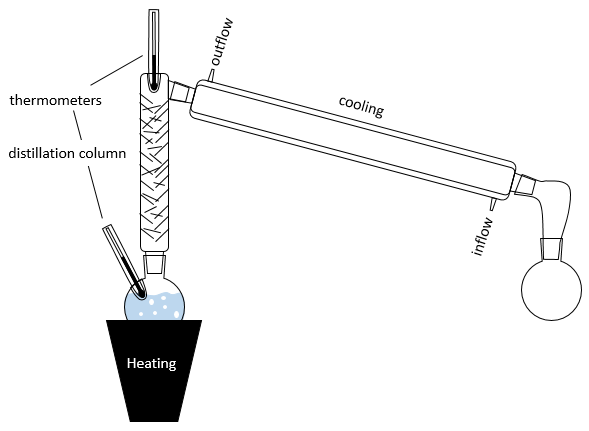The cooling device is composed of two tubes, one thinner placed inside the other one. The inner tube has openings at both extremities and is connected to the column and the collecting balloon. The other tube has lateral openings connected to a counter flow of cool water. The flow of cool water goes this way to avoid an unfilled area and also because the water near the column, that has been heated by the vapour, is quickly evacuated. If the water was going in the opposite direction, the water gets heated by the vapour and the cooling power of the tube would be decreased.

The distillation column if filled with small pieces/tubes of glass to increase to surface of contact with the vapour. In some columns, needles are part of the column and no additional pieces have to be added.

In the distillation column, hot vapour goes up and meets the pieces of glass of lower temperature. At their contact, there is an exchange of heat and the vapour may turn back into liquid, not with the composition of the liquid in the balloon χ0 (to keep the notation of the figure above), but the composition of the vapour χ1. Droplets will eventually fall back into the balloon or evaporate on their way because of the hot vapour. Their composition will now be χ2, as if two distillations had been performed. The vapour continues its way up to the top of the column, getting more pure, and enters the cooling tube where it turns into liquid, finishing its path inside the collecting balloon.

It is good to put some pumice stones in the balloon to avoid overheating and to obtain bubbles of a controled size.

Azeotropes

The diagram of boiling is not always that simple. Some mixtures are said to be azeotrope. An azeotrope mixture acts like a one-component solution, i.e. the mole fraction does not change during the boiling. On the diagram, the curves look like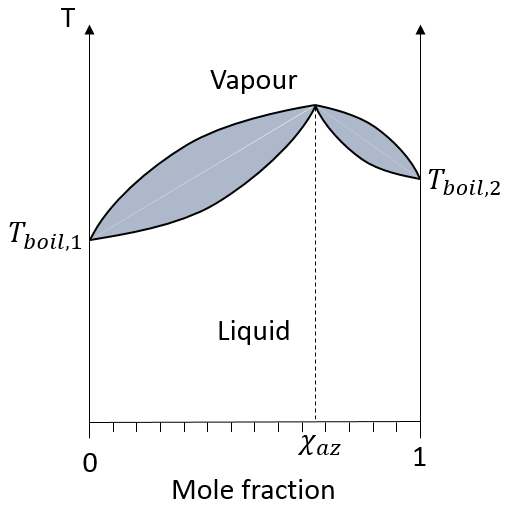If the initial solution has the azeotrope composition, the liquid and vapour compositions are identical and do not change over time. If the initial solution has a smaller mole fraction, we will eventually obtain a pure solution of the solute 1 but it is impossible to obtain a pure solution of the solute 2: the composition tends towards the azeotrope. The same is true if the initial composition of the mixture has a larger mole fraction than χaz: it will be impossible to obtain a pure solution of the solute 1 in this case.

Two types of azeotropes can be sorted: the positive ones and the negative ones. The differences is simply their boiling temperature. On the diagram above, a positive azeotrope is represented: its boiling temperature is larger than the ones of the pure components of the mixture. A negative azeotrope has a lower boiling temperature.

Demixing

In mixtures, different interactions exist: the interactions between two identical molecules and between the different molecules of the mixture. If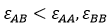then the mixture tends to separate. The expression above means that the interactions between identical molecules are more favourable than the interactions between different molecules. Imagine that in that case the molecules A and B are perfectly mixed together. The interactions between A and B are not necessarily unfavourable but with the Brownian motion, the molecules will tend to regroup between species and they will form clusters/bubbles to have the maximum amount of favourable interactions. That is how emulsions form. The clusters will merge together over time to improve the ratio volume/surface of the clusters and the solution will eventually end as two separated phases. It is the phenomenon of demixing.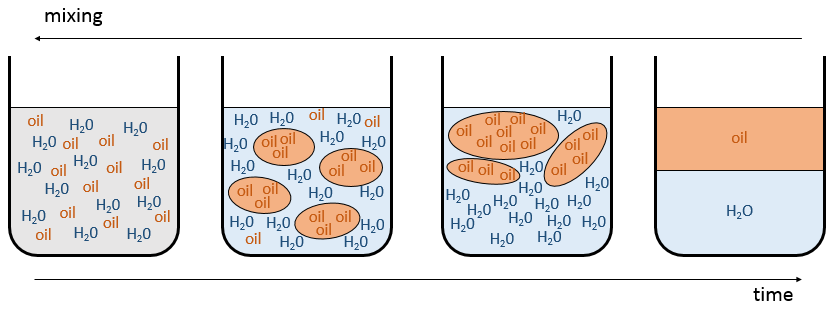For example, oil and water do not mix well. They form two separate phases. Yet, we can dilute a bit of water in oil or vice versa but if we continue to add water, the phases will separate. It is shown on the following figure.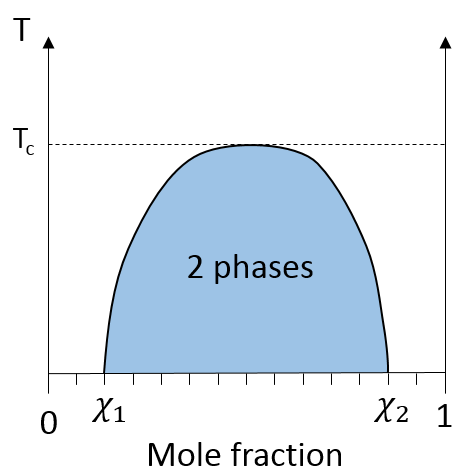If the oil is the component 1 and water the component 2, this figure shows that we can add water to oil up to the mole fraction χ1 before a separation of phases. We can add oil into water without demixing for mole fractions larger than χ2. As we can see, the demixing process depends on the temperature. The quantity of solute that we can dissolve before the demixing depends on the local temperature. Above a critical temperature (not the same as in the phase diagram), the mixture will remain as one phase no matter the composition.

Colligative properties

The colligative properties are properties that depend on the number of the particles in the solvent, of the solvent but not of the solute species. The fact that the boiling temperature of water increases when we add a bit of salt inside is a colligative property. It does not matter that it is salt or something else. The temperature of freezing decreases as well: we use salt on snow to make it melt. In the phase diagram, the coexistence curve has been translated down because of the addition of the salt. The ebullioscopic law says that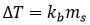With kb the ebullioscopic constant and ms the molality of the solute. When we add salt into water, we add two species: the ions Cl and Na+. It is thus better to write this relation as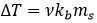ν is called the van’t Hoff factor and is the number of ions found by unit formula. In fact, we observe that this factor is often smaller than its expected value: some of the molecules don’t dissolve in the solution.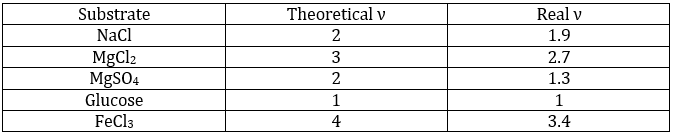That means that for 100 molecules of NaCl in solution, 90 will dissolve to produce the ions Cl and Na+ and 10 molecules don’t dissolve (making a total of 190 molecules instead of 200). ν can be determined from the constant of dissociation of the molecule. For example, all the molecules of a weak acid don’t dissociate in water.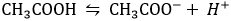The Ka of this reaction is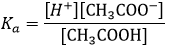Let’s take a look at the concentration of the species before and after the reaction. In fact, instead of the concentrations, we will consider the concentrations/initial concentration of CH3COOH.The total number of molecules is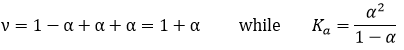So, we know that there is a decrease of temperature due to the colligative properties. The variation of temperature is coupled to a variation of the vapour pressure of the mixture. Intuitively, the vapour pressure of the dissolved salt is in general very small with regards to the vapour pressure of the water. Some salts are not volatile at all. The pressure is equal to the sum of the partial pressures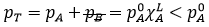The addition of a salt B into the solution A has thus lowered the pressure.

The mathematical demonstration follows:

Considering the dissolution of NaCl in water, the variation of pressure is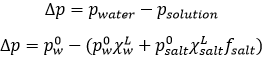Fsalt is a coefficient of activity of the salt, present for reasons similar to that of the van’t Hoff factor ν.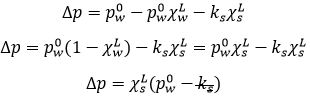We can still simplify the expression: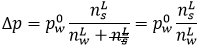On the phase diagram, the coexistence line is translated downwards by Δp. The ΔT can also be calculated: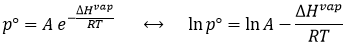The derivative gives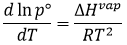Math tells us that it also gives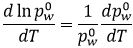So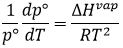dp°/dT is in fact equivalent to Δp/ΔT from the phase diagram. We obtain that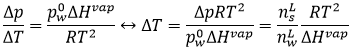The difference of temperature is thus proportional to the square of the temperature and directly proportional to the amount of species in the solvent.

Similarly, it is possible to detect if solids are pure. If a solid melts at a temperature lower than its normal melting temperature, it shows that there are impurities in the solid.

The osmotic pressure is also a colligative property: in cells, the concentration of ions must be identical at each side of the cell membrane. Otherwise the cell expends or shrinks. The osmotic pressure of a solution is the difference in pressure between a solution containing solute particles and the pure liquid solvent when the two are in equilibrium across a semipermeable membrane, i.e. a membrane which allows the passage of solvent molecules but not of solute particles. If the two phases are at the same initial pressure, there is a net transfer of solvent across the membrane into the solution known as osmosis. The process stops and equilibrium is attained when the pressure difference equals the osmotic pressure.

Solubility

The solubility is the ability of a substrate to dissolve in a liquid. We are talking of solubility for solids, salts for instance, and for gases.

Solubility of gases

Some unnaturally sparkling mineral water sold in the market are enriched in carbonic gas. A given quantity of gas can be dissolved in a liquid by the direct injection of the gas into the liquid with a given flow. This quantity is not infinite and depends on the conditions of pressure and of temperature.  If a large pressure is applied, more gas can dissolve in the liquid. The quantity is proportional to the vapour pressure p° of the gas.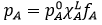fA is a function that depends on the molecule. The molar fraction of A in the solution is thus proportional to the pressure of the gas multiplied by a constant kA=p°A fA. We can rewrite this in function of the concentration in the solution instead of the molar fraction as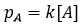A way to dissolve a gas inside a liquid is thus to increase the pressure of the gas.

Acidification of the oceans

We often hear about the greenhouse effect and about the massive impact that the modification of the concentrations of the greenhouse gases may have on our lives.  An important actor of the greenhouse effect is the CO2. Animals exhale CO2 and ours industries and locomotion middles (cars, trains, planes, etc) also produce a lot of this gas. Plants are consuming the CO2 to produce O2 that we breathe. It is a fragile equilibrium that we are menacing with the deforestation, but it is not our point here. The point is that oceans represent a colossal volume of water in which CO2 may be absorbed (each day, it absorbs 4kg of CO2/man, 25 billion kg CO2/year). In the oceans, algae’s are playing the same role than the plants on the ground and their effect is probably as important with regard to the quantities of CO2 absorbed. A global increase of the concentration of CO2 would increase the quantity of CO2 in the oceans, forming carbonic acid (H2CO3) that acidifies the water, slowly menacing the lives of oceanic species, including algae’s, planktons, corals, etc. Before the industrial revolution, the pH of oceans was 8.2. The actual pH is 8.05 and is expected to decrease to 7.8 at the end of this century.

Another way is to change the temperature.  At the beginning of this chapter, we discussed about the free enthalpy of Gibbs G=H-TS. The temperature is thus coupled with the entropy. The ΔG associated with the transition of phase is thus directly dependant on the temperature multiplied by the variation of entropy due to the transition of phase. The dissolution of a gas in a liquid decreases the entropy of the system: the disorder is greater in a gas where there is a lot of space to move and barely no interactions between the molecules than in a liquid where the space is limited and interactions may be strong. On the other hand, the dissolution is exothermic: new liaisons are generated. As a result, there is a competition between the entropy and the enthalpy of the dissolution. The enthalpy can be expressed by ΔH=-RTlnχL2, from the van’t Hoff equation ln Csat = -DH/(RT) + Const.

We can visualise the dissolution of a gas in a liquid with this diagram: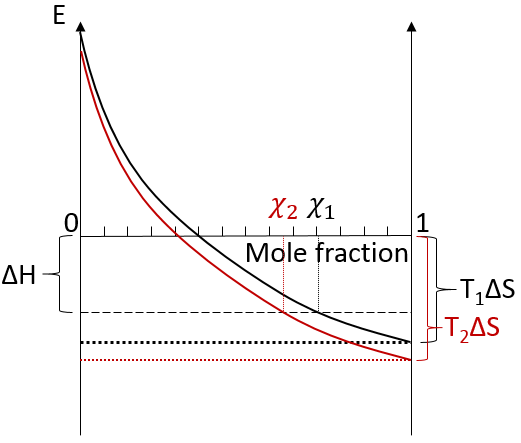The energy is put as ordinate and the mole fraction of the gas in the liquid is put as abscise. A horizontal dashed line is traced at E=ΔH° and two dotted lines at E=TΔS, T1<T2. We will call those lines the enthalpic and the entropic lines. Those lines are in the negative values of the energy as explained before. Next, we trace the curve ΔG(χL2)=TΔS- RTln χL2. This curve has been plotted for two temperatures. The curves terminate for χL2=1 at E=TΔS, i.e. it crosses the entropic line. On its path, the curve crosses the enthalpic line. At the crossing point, we find the maximum amount of gas χ1 that the liquid can dissolve. For larger temperatures (red lines), the entropic line moves deeper in the negative values of E. The curve of ΔG translates the same way, still ending at E=TΔS. ΔH doesn’t change. The crossing point between ΔG and ΔH indicates now a smaller mole fraction of gas χ2 into the liquid. An increase of temperature involves thus a decrease of the solubility of the gas. As a reminder from the phase diagram and the curve of coexistence, the heating of a liquid increases the kinetic energy of the molecules and molecules with a kinetic energy large enough become gaseous.

Solubility of solids

Salts dissolve into ions in polar liquids such as water.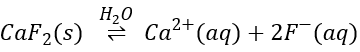In opposition to the solubility of gases, when solids dissolve in water, the entropy increases slightly: first, solutions are ordered (short range order) but solids are more ordered than them. This variation of entropy is small with regards to the variation of entropy of the dissolution of gases. Second, there are more molecules as one molecule of salt gives several ions. Also, the dissolution is endothermic: liaisons are broken to form the ions. Both ΔH and TΔS are thus positive. We determine the quantity of salt that can be dissolved the same way as for the gases. Now that the enthalpic and the entropic lines are in the positive energies, if the temperature increases, the solubility increases.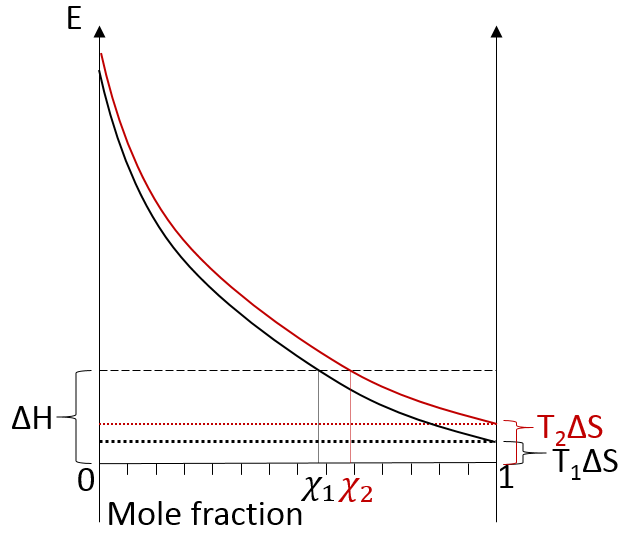Some salts have a negative enthalpy. In this case, the enthalpic line is under the entropic lines whatever the temperature.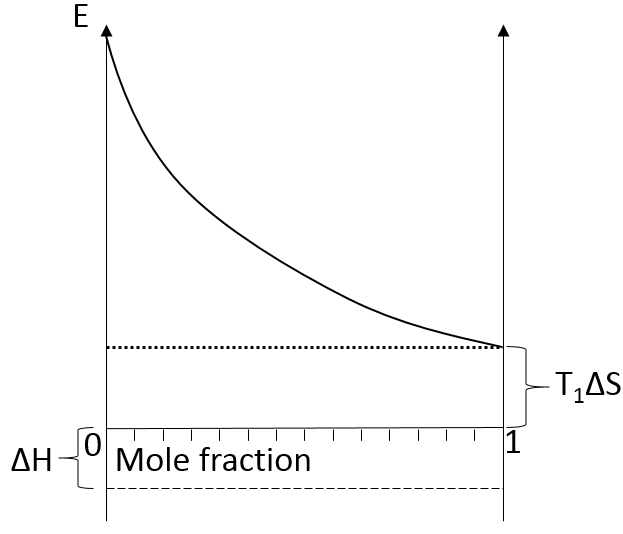There is thus no crossing point between the curve of ΔG and ΔH. The salt is said to be infinitely soluble in this case.

Constant of solubility Ks

The dissolution of a solid inside a solution respects the rules of a chemical reaction: the stoichiometry is respected and the reaction has a constant of reaction defining the speed at which the process takes place.  Let’s define s as the maximum concentration of the salt that can be dissolved. If we put more solid in the solution, crystals will remain (in general) in the bottom of the solution.

In this situation, the concentration of the ions in solution can directly be related to s. For instance, the dissolution of AgCl into water leads to the following equation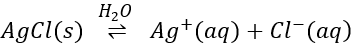With the constant of solubility Ks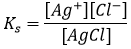As the solid is pure, its concentration is considered as equal to 1 in the equation. It is the case for any solid. When no more salt can be dissociated, the concentration of both ions Ag+ and Cl are equal to s.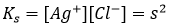s is thus equal to √Ks. For CaF2, three ions are formed during the process.The constant of solubility Ks is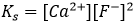In this second case, there are two anions F in solution for each dissolved CaF2. Its concentration won’t be s but 2s.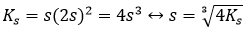As a general expression,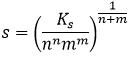With m and n the charge of the ions, or their stoichiometric coefficients (it is equivalent).

The value of Ks gives us precious information on the solubility of a salt, and on how much solid we can put in solution without waste. Remember that Ks depends on the temperature and that it is sometimes necessary to heat a solution to dissolve a precipitate, to reach a given concentration of ions.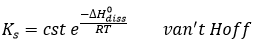This relation is very similar to the expression (Clausius-Clapeyron) for the vapour pressure in the system at the equilibrium p° which was seen at the beginning of this chapter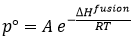It is thus again possible to plot the evolution of Ks or of s with regard to the temperature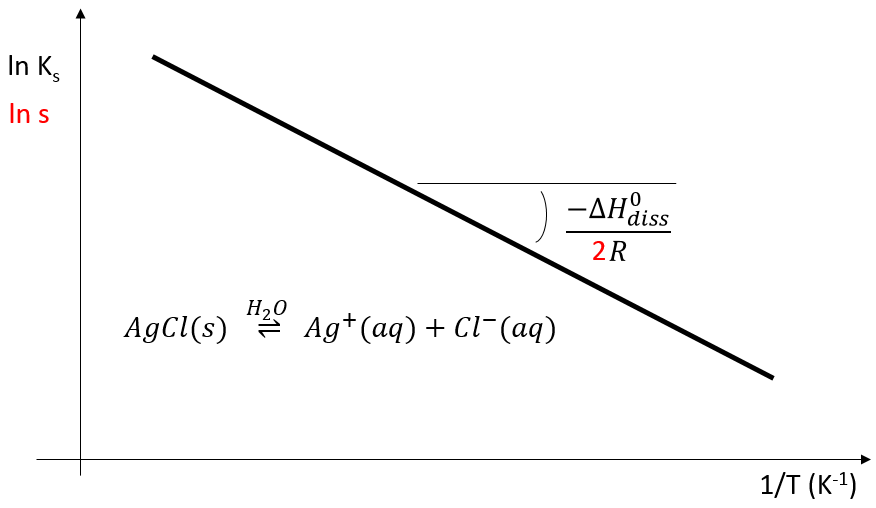The 2 under the fraction bar is the exponent of s in the relation between Ks and s.

Exercises

1.  What is the temperature of ebullition of water at 0.7atm? At 2 atm? (ΔHwvap=40kJ/mol)

2.  Determine the vapour pressure of nitrogen at T=-120°C knowing that its usual ebullition point is -196°C and that ΔHvap=5.6kJ/mol.

3.

The following table shows some experimental measurements of the vapour pressure of the benzene as a function of the temperature.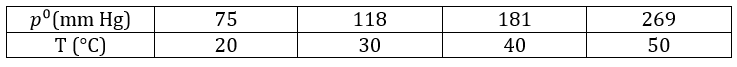Determine the enthalpy of vaporisation of the benzene. If a sample of benzene at T=50°C and p=150mm Hg is cooled down to 40°C in a closed recipient, will it condense? At 30°C? Estimate the temperature at which the gas stats to condensate.

4.  In one mole of ice, there are two moles of H bonds. Determine the energy of the H bonds in one mole of ice if you know that at the triple point the enthalpies of fusion and vaporisation are respectively 333.9J/g and 2493.7J/g. Can you estimate the amount of H bonds in the water?

5.  In the following systems, how many phases, independent components and variance?

1. water in equilibrium with its vapour at 1 atm
2. water in equilibrium with its vapour

6.  1l of naphthalene C10H8 in benzene C6H6 has 67g of solute in it. Give the mass fraction, molar fraction, molarity and the molality in naphthalene if the density of the solution is equal to 0.850.

7.  Which mass of decahydrated carbonate of sodium do you have to weight to prepare 100g of a solution of Na2CO3 10%, knowing that the density of the solution will be 1.10. Determine the molarity, molality and molar fraction in Na2CO3.

8.  We mix together 30ml of n-heptane (ρ=0.684) and 20ml of n-octane (ρ =0.703). Their vapour tensions at 100°C are respectively 829 and 344torr. Find out the molar fraction of the solution and of the vapour if the temperature is 100°C, Is this an ideal solution? Draw the corresponding isotherm (T=100°C) diagram of phases of this melange.

9.  The temperature of ebullition of the benzene and of the toluene are respectively 80.1°C and 110.6°C. Their enthalpies of vaporisation are 33.9kJ/mol and 37.4kJ/mol. Determine the vapour pressure of those two compounds at 90°C and 100°C.

Determine the composition of the solution of benzene and toluene that starts to boil at those temperatures if the pressure is 1 atm.

What is the composition of the vapour at those temperatures?

10.  How much ethylene glycol (CH2OH)2 has to be dissolved in 2l of water to prevent it to freeze at -10°C considering a constant of freezing Kf=1.86K kg mol-1 for the water)? Same question with CaCl2 instead of the ethylene glycol, considering a total dissociation of the salt.

11.  The osmotic pressure of 100ml of a solution containing 0.947g of a protein corresponds to a column of water of 12mm at 25°C. What is the molar mass of the protein?

1.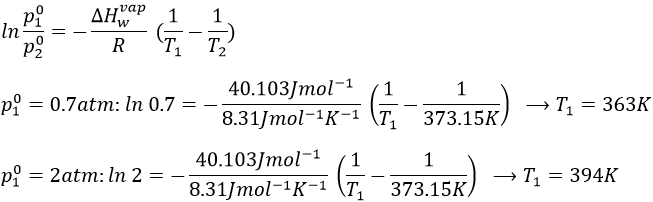2.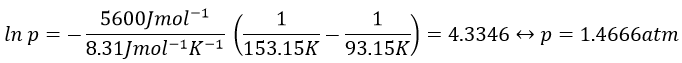3.  If we plot ln p0 as a function of 1/T, the slope α equals –ΔHvap/R, so ΔHvap=- αR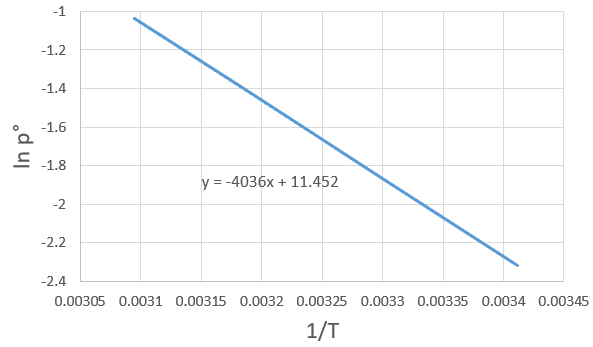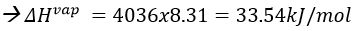The sample is not at the equilibrium but we have the values of the vapour pressure at the equilibrium. At 40°C, the vapour pressure is larger than the pressure in the flask, so the gas doesn’t condense. At 30°C however, the vapour pressure from the table is smaller than the pressure in the flask and the gas condense under the effect of the cold.

To estimate the temperature at which the gas starts to condensate, we chose one value of the table and apply the formula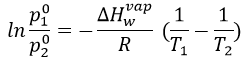to determine the temperature at which the condensation begins: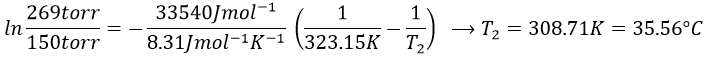4.  At the triple point, the chemical potential are equals. It means that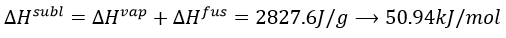This enthalpy is the energy required to break the bonds between the molecules, i.e. the hydrogen bonds. As there are two H bonds per mol, the energy of one mol of H bond is 25,57kJ.

We can estimate the amount of H bonds in the water from the enthalpy of fusion. During the fusion, a given amount of H bonds are broken to make the solid ice become liquid.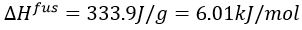We know that 25.57kJ are required to break 1 mol of H bond, so we can estimate that 6.01/25.57=0.235 mol are broken during the fusion. There are thus 1.765 mol of H bond/mol of water.

5.  The expression for the variance is V=2+c-φ-n where c is the amount of constituents, φ is the amount of phases and n is the amount of fixed parameters.

1. V=2+1-2-1=0
2. V=2+1-2-0=1

6.  The density is the mass by unit of volume, so the litre of solution weights 850g. We have 67g of naphthalene and 783g of benzene. The mass fractions are thus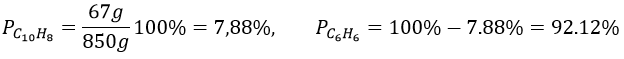Knowing the masses, we can find the quantity of moles for both species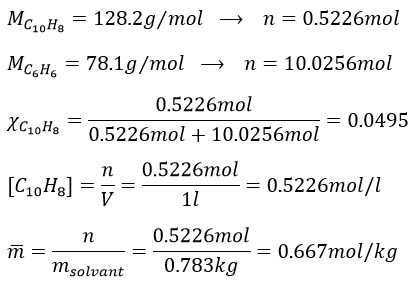7.  As the mass of the solution is 100g, we want to have 10g of Na2CO3. To determine the mass of decahydrated carbonate, we first need to know the amount of moles of Na2CO3 in solution.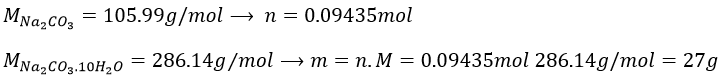The volume of solution is 100g/1.1g ml-1=90.90..90..ml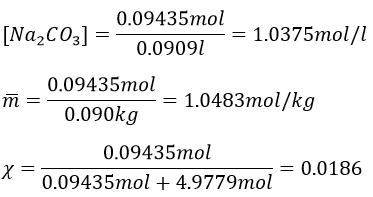In the vapour, the molar fractions are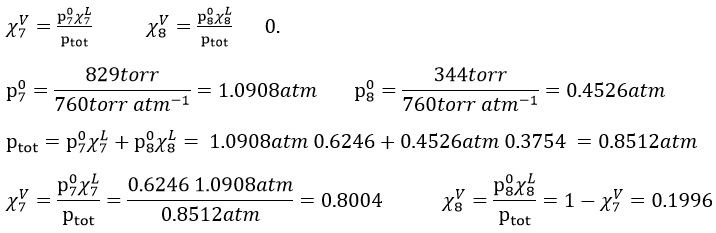This melange is an ideal solution because the n-heptane and the n-octane are both nonpolar species without dipolar moment and unable to form H bonds. As a result, they don’t have any specific interactions together.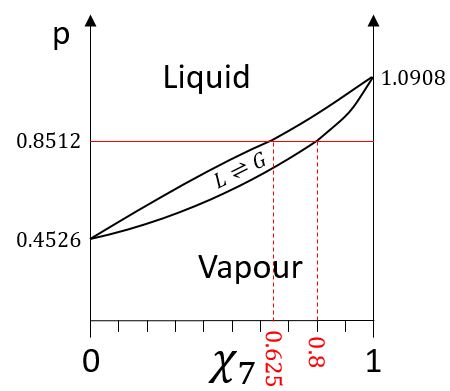The information we have to draw the phases diagram are that the pure solutions have a vapour tension of 0.4526atm (χ7=0) and 1.0908atm (χ7=1). We also know that at p=0.8512atm, the molar fraction is 0.625 for the liquid and 0.8 for the gas.

8.  The partial pressures can be found with the equations of Clausus-Clapeyron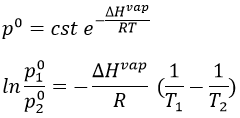Either we find the constant in the first one with the values we know from the wording to be able to solve the equations at the given temperatures. Or we can use the second equation that doesn’t need to determine the value of the constant.

First method: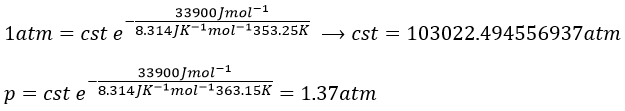Second method: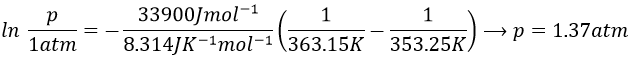We do the same for the tow compounds and the two temperatures to find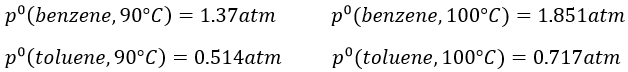To determine the molar fractions at a given temperature, we use this expression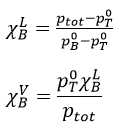At 90°C, we have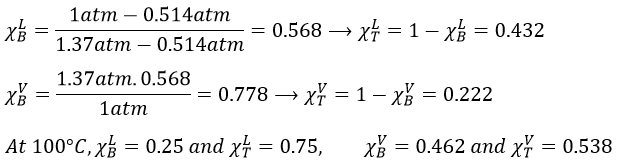We can plot all those points on the phase diagram.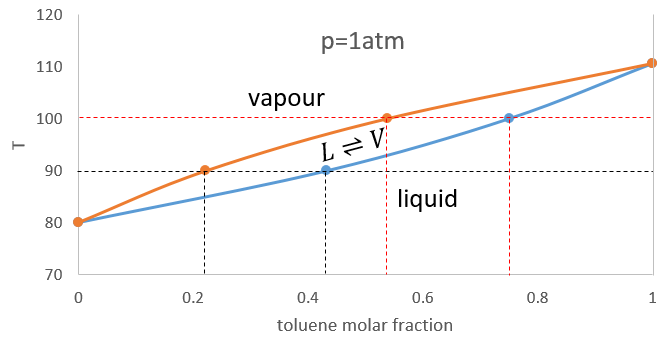9.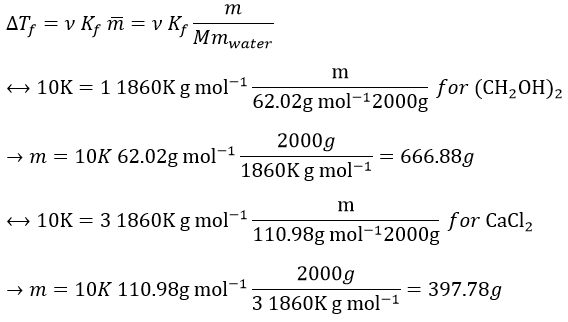10.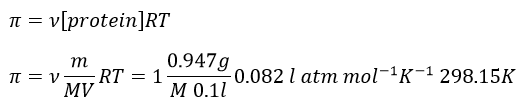The osmotic pressure is normally given as a pressure. To obtain it in atm, we transform the height of water in height of mercury and then in atm. The ratio between the density of mercury and water is 13.59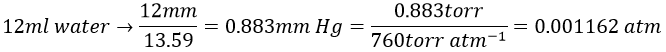We can now determine the molar mass of the protein